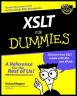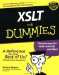# Numerically SpeakingChapter 11 - XPath Data Types and Functions XSLT For Dummies by Richard Wagner Hungry Minds 2002Although XSLT wasnt designed for number crunching , you can use it to do some basic manipulation of numbers . Check out the following built-in functions.

### Converting an object into a number

You can use the number() function to convert the specified object into a number. If the object is a string, then it is converted to the nearest numeric value specified by the string. A boolean value of false is converted to , while true returns a 1 . A node is first converted to a string value and then is converted as a string. The functions syntax is:

`  number  number([object]) `

The following snippet of XSLT converts a string to a number for calculation:

` <xsl:value-of select="3.34*number('4.5')"/> `

Results in:

` 15.03 `

### Rounding numbers

You can round numbers to the nearest integer number with the round() function:

`  number  round(number) `

By applying round() to the example in the preceding section:

` <xsl:value-of select="round(3.34*number('4.5'))"/> `

I get a rounded integer value:

` 15 `

### Getting highest and lowest integer values

You can obtain the highest and lowest integer values by using the floor() and ceiling() functions:

`  number  floor(number)  number  ceiling(number) `

When called, floor() evaluates the specified number value and returns the lowest integer number that is not less than the value. The ceiling() function does the converse returning the highest integer number that is not greater than the value. To see how this works, take a look at the following code snippet:

` Floor: <xsl:value-of select="floor(3230.20)"/> Ceiling: <xsl:value-of select="ceiling(3230.20)"/> `

The results look like this:

` Floor: 3230 Ceiling: 3231 `

### Summing it all up

The sum() function allows you to get the sum of the number values of the nodes in the specified nodeset:

`  number  sum(nodeset) `

In other words, sum() first converts each node in the nodeset to a number and then tallies up the numbers.

The following template rule uses sum() to add up the numeric values for all the fatgrams elements in the source tree:

` <xsl:template match="/"> If you eat all of our entrees, you will eat a total of <xsl:value-of select="sum(//fatgrams)"/> grams of fat. </xsl:template> `

The following sentence results from the transformation:

` If you eat all of our entrees, you will eat a total of 138 grams of fat. `

### Formatting a number

When numbers are displayed as strings, youll often want to format them in a specific manner. The format-number() function allows you to take a number and obtain a string version of it in the format you specify. Its syntax is:

`  string  format-number(number, formatstring [, decimalformat]) `

When processed , the number argument is converted to a string and is formatted based on formatstring . Table 11-1 shows the symbols you can use to compose the formatstring value.

Table 11-1: formatstring Symbols

Symbol

Means Example (using 1,000 as the number to be formatted)

Any numeric digit

With 00000, 1,000 would display as 01000

#

A digit with zero showing as absent

With #####, 1,000 would display as 1000

.

A decimal point in a number

With #####.00, 1,000 would display as 1000.00

,

A thousands grouping symbol

With #,####, 1,000 would display as 1,000

-

Default negative prefix

With -####, 1,000 would display as -1,000

%

Multiply by 100 and show as percentage

With ####%, 1,000 would display as 100000%

\$

U.S. currency sign ( replaced by appropriate currency symbol)

With \$####, 1,000 would display as \$1000

X

Any other characters can be used in the prefix or suffix

With ABC-####, 1,000 would display as ABC-1000

Technical Stuff   The formatstring argument is based on the DecimalFormat class of Java 1.1. For full details, go to java.sun.com/products/jdk/1.1/docs/api/java.text.DecimalFormat.html .

The following template rule shows how the format-number() function can output a variety of numeric formats based on the formatstring argument:

` <xsl:template match="/"> <xsl:variable name="constant" select="00433339.03"/> <xsl:value-of select="format-number(\$constant, '#')"/><xsl:text> </xsl:text> <xsl:value-of select="format-number(\$constant, '00000000000')"/><xsl:text> </xsl:text> <xsl:value-of select="format-number(\$constant, '#,###')"/><xsl:text> </xsl:text> <xsl:value-of select="format-number(\$constant, '#.##')"/><xsl:text> </xsl:text> <xsl:value-of select="format-number(\$constant, '\$#,###,###.##')"/><xsl:text> </xsl:text> <xsl:value-of select="format-number(\$constant, '#%')"/><xsl:text> </xsl:text> <xsl:value-of select="format-number(\$constant, 'SE-#-EP')"/><xsl:text> </xsl:text> </xsl:template> `

The results are shown below:

` 433339 00000433339 433,339 433339.03 3,339.03 43333903% SE-433339-EP `2000-2002    FeedbackXSLT for Dummies
ISBN: 0764536516
EAN: 2147483647
Year: 2002
Pages: 148

Similar book on Amazon

flylib.com © 2008-2017.
If you may any questions please contact us: flylib@qtcs.net